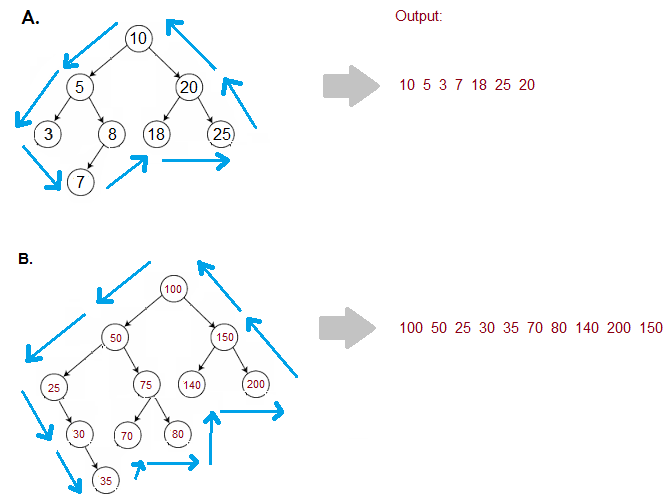New update is available. Click here to update.

# Boundary Traversal of Binary Tree

Last Updated: 9 Oct, 2020
Difficulty: Hard

## PROBLEM STATEMENT

#### You have been given a binary tree of integers. Your task is to print the boundary nodes of this binary tree in Anti-Clockwise direction starting from the root node.

##### NOTE:
``````The boundary nodes of a binary tree include nodes from the left boundary, right boundary and the leaf nodes without duplicate nodes. However, the values from the nodes may contain duplicates.
``````
##### For Example:##### Input Format:
``````The first line contains an integer 'T' which denotes the number of test cases or queries to be run. Then the test cases follow.

The only line of each test case contains elements in the level order form. The line consists of values of nodes separated by a single space. In case a node is null, we take -1 on its place.

For example, the input for the tree depicted in the below image will be:
````````````1
2 3
4 -1 5 6
-1 7 -1 -1 -1 -1
-1 -1
``````

#### Explanation :

``````Level 1 :
The root node of the tree is 1

Level 2 :
Left child of 1 = 2
Right child of 1 = 3

Level 3 :
Left child of 2 = 4
Right child of 2 = null (-1)
Left child of 3 = 5
Right child of 3 = 6

Level 4 :
Left child of 4 = null (-1)
Right child of 4 = 7
Left child of 5 = null (-1)
Right child of 5 = null (-1)
Left child of 6 = null (-1)
Right child of 6 = null (-1)

Level 5 :
Left child of 7 = null (-1)
Right child of 7 = null (-1)

The first not-null node(of the previous level) is treated as the parent of the first two nodes of the current level. The second not-null node (of the previous level) is treated as the parent node for the next two nodes of the current level and so on.
The input ends when all nodes at the last level are null(-1).
``````
##### Note :
``````The above format was just to provide clarity on how the input is formed for a given tree.
The sequence will be put together in a single line separated by a single space. Hence, for the above-depicted tree, the input will be given as:

1 2 3 4 -1 5 6 -1 7 -1 -1 -1 -1 -1 -1
``````
##### Output Format:
``````For each test case, print the boundary nodes of the given binary tree separated by single spaces.

Print the output of each test case in a separate line.
``````
##### Note:
``````You are not required to print the expected output, it has already been taken care of. Just implement the function.
``````
##### Constraints:
``````1 <= T <= 10^2
1 <= N <= 2^12

Where 'N' is the total number of nodes in the binary tree.

Time Limit: 1 sec
``````